# Comparing Graphs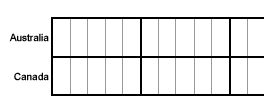In this unit, we learned about line, bar and circle graphs. We learned how to read and interpret information from each type of graph, and how to construct these graphs. If you were asked to create a graph from a given set of data, how would you know which type of graph to use? Which graph we choose depends on the type of data given and what we are asked to convey to the reader. The information below will help you determine which type of graph to use.

Tables are used to organize exact amounts of data and to display numerical information. Tables do not show visual comparisons. As a result, tables take longer to read and understand. It is more difficult to examine overall trends and make comparisons with tables, than it is with graphs.

Line graphs are used to display data or information that changes continuously over time. Line graphs allow us to see overall trends such as an increase or decrease in data over time.

Bar graphs are used to compare facts. The bars provide a visual display for comparing quantities in different categories or groups. Bar graphs help us to see relationships quickly. However, bar graphs can be difficult to read accurately. A change in the scale in a bar graph may alter one's visual perception of the data.

Circle Graphs are used to compare the parts of a whole. Circle graphs represent data visually in the same proportion as the numerical data in a table: The area of each sector in a circle graph is in the same proportion to the whole circle as each item is to the total value in the table. Constructing an accurate circle graph is difficult to do without a computer. This is because you must first find each part of the whole through several elaborate calculations and then use a protractor to draw each angle. This leaves a lot of room for human error. Circle graphs are best used for displaying data when there are no more than five or six sectors, and when the values of each sector are different. Otherwise they can be difficult to read and understand.

Problem 1: The table below shows the number of sneakers sold by brand for this month. Construct a graph which best demonstrates the sales of each brand.

 Sneakers Sold This Month Brand Number Sold Adidas 25 New Balance 18 Nike 32 Reebok 15 Other 10

Analysis: The numerical data in this table is not changing over time. So a line graph would not be appropriate for summarizing the given data. Let's draw a circle graph and a bar graph, and then compare them to see which one makes sense for this data. Before we can draw a circle graph, we need to do some calculations. We must also order the data from greatest to least so that the sectors of the circle graph are drawn from largest to smallest, in a clockwise direction.

 Sneakers Sold This Month Brand Number Sold Percent Decimal Angle Measure Nike 32 32 0.32 0.32 x 360° = 115.2° Adidas 25 25 0.25 0.25 x 360° = 90° New Balance 18 18 0.18 0.18 x 360° = 64.8° Reebok 15 15 0.15 0.15 x 360° = 54° Other 10 10 0.10 0.10 x 360° = 36° Total 100 100% 1.00 = 1 360°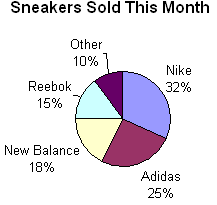Circle graphs are best used to compare the parts of a whole.

The circle graph above shows the entire amount sold. It also shows each brand's sales as part of that whole.

The circle graph uses the total of all items in the table.

Each sector of the circle graph is in the same proportion to the whole circle as the number of sales for that industry is to the entire amount of sales from the table.

To construct an accurate circle graph, you must first order the data in the table from greatest to least. You also need to find each part of the whole through several elaborate calculations and then use a protractor to draw each angle.

If we were asked to show that the Nike brand dominates the sneaker industry, then the circle graph would be a better choice for summarizing this data.Bar graphs are used to compare facts.

The bar graph stresses the individual sales of each brand as compared to the others.

The bar graph does not use the total of all items in the table.

The bar graph simply gives a visual listing of the information in the table.

The number of sneakers sold for each item in the table matches the value of each bar in the bar graph. This makes the bar graph a more direct and accurate way of representing the data in the table.

We were asked to construct a graph which best demonstrates the sales of each brand.

Solution: Each graph above has its own strengths and limitations. However, the bar graph is the best choice for summarizing this data based on what we were asked to convey to the reader.

Problem 2: The table below shows the humidity level, recorded in Small Town, NY for seven days. Construct a graph which best demonstrates the humidity level for each day.

 Humidity Levels in Small Town, NY Day Humidity Level (%) 1 51 2 59 3 65 4 68 5 70 6 67 7 72

Analysis: The humidity level is given as a percent. At first glance, this might lead one to think that a circle graph should be used to summarize this data. However, the data in the table does not indicate any parts in relation to a whole. Thus, a circle graph is not the right choice. The data in this table is changing over time. So a line graph would be the appropriate choice for summarizing the given data.

Solution: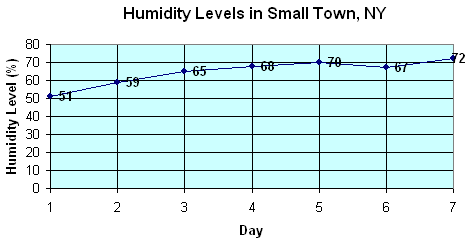Problem 3: The table below shows the composition of Earth's atmosphere. Construct a graph which best represents the composition of the Earth's Atmosphere.

 Composition of Earth's Atmosphere Gas Percent Nitrogen 77 Oxygen 21 Other 2

Analysis: The word composition indicates that we are looking at the parts of a whole. The Earth's Atmosphere is the whole (100%) and each gas is a part of that whole. Accordingly, a circle graph is the best choice for summarizing this data.

Solution: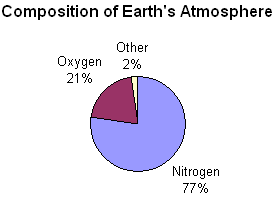Problem 4: The table below shows the surface area of each continent in square kilometers. Construct a graph which best represents this data.

 Surface Area of Continents Continent Surface Area (km2) Africa 15,000,000 Antarctica 14,200,000 Asia 44,936,000 Australia 7,614,500 Europe 10,525,000 North America 23,500,000 South America 17,819,100

Analysis: The numerical data in this table is not changing over time. So a line graph would not be appropriate choice for summarizing the given data. Let's draw a circle graph and a bar graph and compare them to see which one makes sense for this data. Before we can draw a circle graph, we need to do some calculations. We must also order the data from greatest to least so that the sectors of the circle graph are drawn from largest to smallest in a clockwise direction.

 Surface Area of Continents Continent Surface Area (km2) Percent Decimal Angle Measure Asia 44,936,000 33.64 .3364 .3364 x 360° = 121.104° North America 23,500,000 17.59 .1759 .1759 x 360° = 63.324° South America 17,819,100 13.33 .1333 .1333 x 360° = 47.988° Africa 15,000,000 11.23 .1133 .1133 x 360° = 40.788° Antarctica 14,200,000 10.63 .1063 .1063 x 360° = 38.268° Europe 10,525,000 7.88 .0788 .0788 x 360° = 28.358° Australia 7,614,500 5.70 .0570 .0570 x 360° = 20.52° Total 133,594,600 100.00 1.00 = 1 360°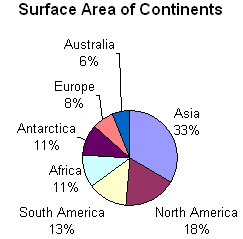Do Africa and Antarctica really have the same surface area? The tool we used to create this circle graph rounded each value to the nearest whole percent. As a result, the circle graph shows that Africa and Antarctica both have a surface area of 11%; whereas the table shows numbers for these continents that are similar, but not the same.

Circle graphs are used to compare the parts of a whole. However, they are best used when there are no more than five or six sectors and when the values of each section are different.

The circle graph above has several sectors with similar sizes. It also has seven sectors. This makes it a bit difficult to compare the parts and to read the graph. However, the world has seven continents, so it does  make sense to use a circle graph to compare the parts (continents) with the whole (world).The bar graph shows the surface area in millions of square kilometers. This was necessary in order to create a bar graph that is not confusing because of too many gridlines. The bar graph shows a surface area of 15.00 million sq. km for Africa and a surface area of 14.20 million sq km. for Antarctica.

Bar graphs are used to compare facts. The bar graph stresses the individual items listed in the table as compared to the others. The bar graph does not show the total of all items in the table.

The bar graph above allows us to compare the surface area of each continent. However, it does not allow us to compare the parts to the whole. So we cannot see the relationship between the surface area of each continent and the surface area of all seven continents.

Solution: It is difficult to determine which type of graph is appropriate for the given data in this problem. Each graph above has its own strengths and limitations. You must choose one of these solutions based on your own judgment.

Summary: Graphs help us examine trends and make comparisons by visually displaying data. Before we can graph a given set of data from a table, we must first determine which type of graph is appropriate for summarizing that data. There are several types of graphs, each with its own purpose, and its own strengths and limitations. Which one we choose depends on the type of data given, and what we are asked to convey to the reader.

### Exercises

Directions: Enter one of the following answers for each exercise below: line, bar or circle. For each exercise below, click once in the ANSWER BOX, type in your answer and then click ENTER. Your answer  should be given as a word or as a whole number. After you click ENTER, a message will appear in the RESULTS BOX to indicate whether your answer is correct or incorrect. To start over, click CLEAR.

 1.The ages of 7 trumpet players in a band are 13, 12, 11, 12, 11, 10 and 12. What type of graph would be appropriate for comparing the ages of these trumpet players? ANSWER BOX:    RESULTS BOX:
 2.The federal hourly minimum wage was recorded each year from 1990 to 2007. What type of graph would best show the changes in minimum wage during this time period?   ANSWER BOX:    RESULTS BOX:
 3.When asked if "antidisestablishmentarianism" has 28 letters, 50 people said yes, 35 people said no and 15 people said I don't know. What type of graph would best compare these responses  to each other and with the total? ANSWER BOX:    RESULTS BOX:
 4.The growth of 7 different plants was recorded in centimeters. What type of graph would be best for comparing the growth of each plant? ANSWER BOX:    RESULTS BOX:
 5.In a city, the rainfall was recorded in inches each month for 12 months. What type of graph would best display the change in rainfall? ANSWER BOX:    RESULTS BOX: